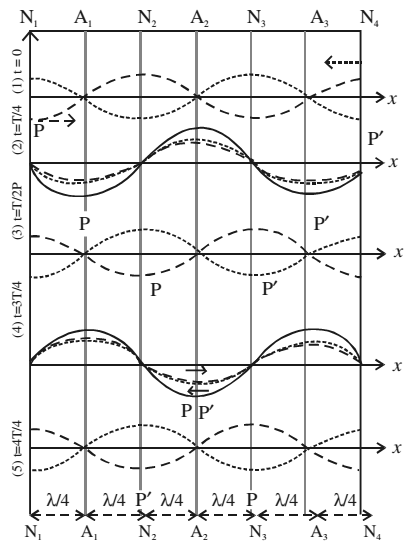# Formation of Stationary (Standing) Waves

Initially, at t = 0, the incident wave and the reflected wave are in the opposite phases. Hence the resultant displacement at each point is zero. All the particles are in their respective mean positions.

At t = T/4 s, the incident wave has advanced to the right by λ/4 and the reflected wave has advanced to the left by λ/4. The resultant displacement at each point is maximum. Hence the particle velocity at each point is zero and the strain is maximum.

At t = T/2 s, the incident wave advances a distance λ/2 to the right and the reflected wave advances a distance λ/2 to the left. At each point, the displacements being in the opposite directions, have a zero resultant.

At t = 3T/4 s, the two waves are again in the same phase. The resultant displacement at each point is maximum. The particle velocity is zero but the strain is maximum possible.

At t = 4T/4 s, the incident and reflected waves at each point are in the opposite phases. The strain ∆y/∆x at each point is zero.At points N1, N2, N3 and N4, the amplitude is zero but the strain is maximum. Such points are called nodes.

At points A1, A2 and A3, the amplitude is maximum but the strain is minimum. These points are called antinodes.

The distance between two successive nodes or between two successive antinode is λ/2. The distance between a node and next antinode is λ/4.

The time period of oscillation of a stationary wave equals the time period of the two travelling waves whose superposition has resulted in the formation of the stationary wave.

The energy alternately surges back and forth about a point but on an average, the energy flow past a point is zero.

Superposition of two identical collinear waves travelling with the same speed in opposite directions leads to formation of stationary waves. They are called stationary waves, because the wave form does not move forward, but alternately shrinks and dilates. The energy merely surges back and forth and on an average, there is no net flow of energy past a point.